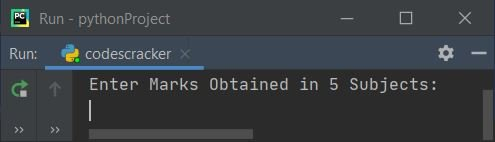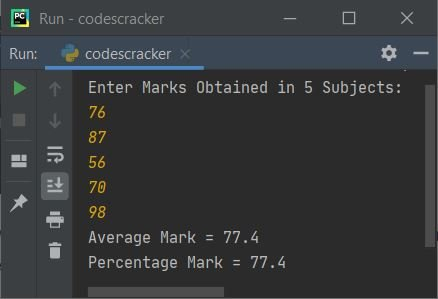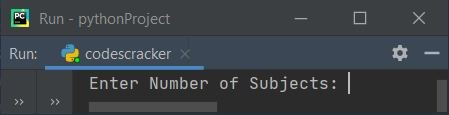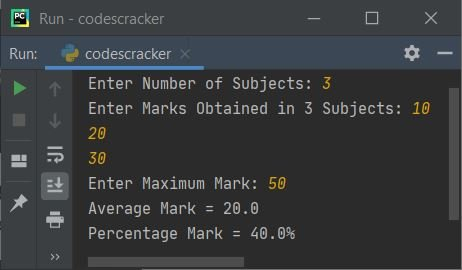# Python Program to Calculate Average and Percentage Marks

In this article, you will learn and get code in Python, to calculate and print average and percentage marks based on marks entered by user at run-time. Here are the list of programs:

• Calculate Average and Percentage Marks based on Marks obtained in 5 Subjects
• Modified Version of above Program. This program uses for loop to receive marks from user
• Allow User to define the number of subjects, maximum mark along with marks obtained in all subjects

## Calculate Average & Percentage Marks in Python

To calculate average and percentage marks in python, you have to ask from user to enter marks obtained in 5 subjects. And then calculate and print average and percentage marks as shown in the program given below:

```print("Enter Marks Obtained in 5 Subjects: ")
mOne = int(input())
mTwo = int(input())
mThree = int(input())
mFour = int(input())
mFive = int(input())

sum = mOne+mTwo+mThree+mFour+mFive
avg = sum/5
perc = (sum/500)*100

print(end="Average Mark = ")
print(avg)
print(end="Percentage Mark = ")
print(perc)```

Here is the sample run of the above Python program, shows how the average and percentage marks of student gets printed on output:The screenshot given above shows the initial output. Now enter marks obtained in 5 subjects one by one and then press `ENTER` key to calculate and print average and percentage marks as shown in the following screenshot:In above program, the following statement:

`print(end="Average Mark = ")`

is used to print Average Mark = without a newline. Because the print() statement automatically prints a newline after printing all the things inside its braces.

Note - The end= ends the line with the thing present inside "" after it. That is, the next thing starts from the same line (in above program).

Note - In above program, if we've to receive marks obtained in 10 subjects, then we've to write the input code again and again 10 number of times. Therefore, let's use the loop to do the same task in dynamic way.

### Receive Marks using for Loop

This program does the same job as of previous program. The only difference is, this program uses for loop to receive the marks obtained in 5 subjects. The for loop also uses to calculate the sum of all subject's mark:

```mark = []
tot = 0
print("Enter Marks Obtained in 5 Subjects: ")
for i in range(5):
mark.insert(i, int(input()))
for i in range(5):
tot = tot + mark[i]

avg = tot/5
perc = (tot/500)*100

print(end="Average Mark = ")
print(avg)
print(end="Percentage Mark = ")
print(perc)```

This program produces the same output as of previous program.

Note - The range() function returns a sequence of values. By default, the value starts with 0 and increments by 1. It stops before a number specified as argument of the function.

Therefore from above program, the following code:

`for i in range(5):`

is used to execute the following statement:

`mark.insert(i, int(input()))`

five times with value of i from 0 to 4. That is, input from user gets received and stored in the list mark[] one by one, in this way:

• First value gets stored in mark
• Second value gets stored in mark
• Third value gets stored in mark
• and so on upto
• Fifth value gets stored in mark

### Get Number of Subject, Obtained Marks, Maximum Mark from User

Now let's create a program that calculates average and percentage marks based on marks obtained in N number of subjects.

This program allows user to provide or define all the things such as total number of subject, marks obtained in all subjects, and maximum mark. After receiving these inputs from user, the program calculate and prints the average and percentage marks:

```mark = []
totMark = 0
print(end="Enter Number of Subjects: ")
totSub = int(input())

print(end="Enter Marks Obtained in " + str(totSub) + " Subjects: ")
for i in range(totSub):
mark.insert(i, int(input()))

print(end="Enter Maximum Mark: ")
maxMark = int(input())

for i in range(totSub):
totMark = totMark + mark[i]
avg = totMark/totSub
perc = (totMark/(totSub*maxMark))*100

print("Average Mark = " + str(avg))
print("Percentage Mark = " + str(perc) + "%")```

Here is its sample run:Now supply inputs say 3 as number of subjects, 10, 20, 30 as marks obtained in 3 subjects, and finally 50 as maximum mark of subject. Here is the sample output based on these user inputs:Note - The str(totSub) is used to convert totSub to string. Because the + operator adds value of same type, otherwise it gives an error.

#### Same Program in Other Languages

Python Online Test

« Previous Program Next Program »